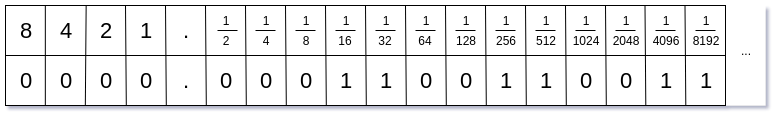# Doubles can be dangerous

10-03-2019

### Introduction

In my career, I’ve heard many times:

“Always use the proper data type.”

or according to mine.Net experience:

“Never use double to math calculations.”

Sounds familiar to you? Do you think it is silly? Do you think you don’t care about it? I assume that you’ve heard similar statements and please don’t think that it’s only claptrap. If you don’t agree with me or you want to know why using doubles is so dangerous this post is made for you.

### Simple experiment

Let’s start with a simple experiment. Of course, you can do it on your own. Take a look at the following code:

``````using System;

namespace Double
{
class Program
{
static void Main(string[] args)
{
double a = 0.1;
double b = 0.2;

if (a + b == 0.3)
{
Console.WriteLine(\$"{a} + {b} is 0.3");
}
else
{
Console.WriteLine(\$"Math is broken. {a} + {b} isn't 0.3. That's odd!?!?");
}
}
}
}
``````

Do you guess how the result will be? Let check this out:

``````rafpie@Oxygen ~/Desktop/double dotnet run
Math is broken. 0.1 + 0.2 isn't 0.3. That's odd!?!?

``````

Our experiment proofs that math is broken. What!?! That isn’t possible. We were taught that 0.1 + 0.2 = 0.3. What the hell is going on here?The crucial point is how our computer represents doubles. I assume that you’re familiar with the number’s binary representation if not here is an article about that. TThe representation of 0.1 in the binary model is as follows:Mostly we focus on the integer part (the left side of the chart), but for now, the right side is more important. To receive 0.1 we have to sum the corresponding powers of two:

1/2^4 + 1/2^5 + 1/2^8 + 1/2^9 + 1/2^12 + 1/2^13+….

Let’s sum first six elements:

1/16 + 1/32 + 1/256 + 1/512 + 1/4096 + 1/8192 =0,099975586

As you can see, we received an approximated result. That leads us to the simple conclusion:

Never compare double values with the == operator!

In the best-case scenario, you will miscalculate the high of your quarterly bonus, in the worst-case scenario someone can die. I’m deadpan here. On February 25, 1991 (the Gulf War) an American Patriot missile battery used double values in the calculation. Due to inaccuracy, 28 soldiers lost their lives. More info you can find here.

### The solution

You can ask how we can overcome this problem. The answer is to use decimal values. The value with the decimal type is stored as a large integer with the decimal point shifted. That means, 0.1 is stored as 1, 0.123 is stored as 123. If you don’t trust me, modify our previous example and change datatype from double to decimal (Notice, you have to add M after the number to indicate the literal decimal value).

``````using System;

namespace Decimal
{
class Program
{
static void Main(string[] args)
{
decimal a = 0.1M;
decimal b = 0.2M;

if (a + b == 0.3M)
{
Console.WriteLine(\$"{a} + {b} is 0.3");
}
else
{
Console.WriteLine(\$"Math is broken. {a} + {b} isn't 0.3. That's odd!?!?");
}
}
}
}
``````

The result will be compatible with the expected one:

``````rafpie@Oxygen ~/Desktop/decimal dotnet run
0.1 + 0.2 is 0.3

``````

Uff, math works.### Summary

I hope that I explained to you why we should be careful with double values. As you can see this could have significant impact correctness of the program’s calculations. I premise on double type built-in the .Net Framework. If someone knows how this problem is addressed in other programming languages, please share that with us.

Note: Background images cames from the pixabay.